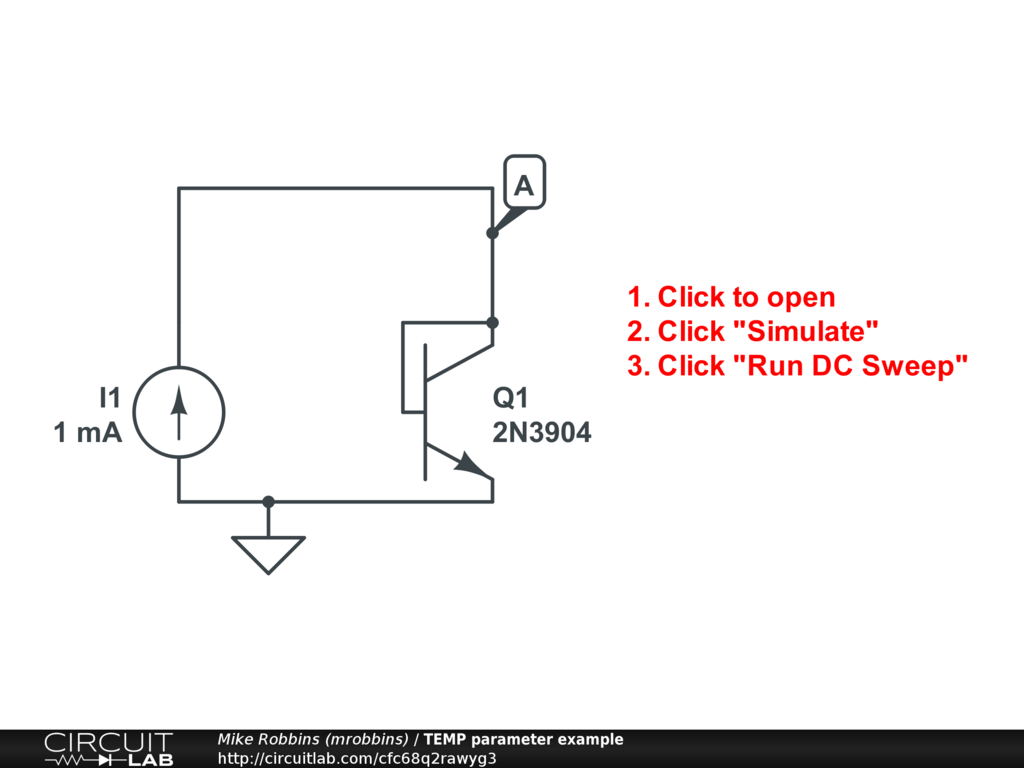## Bug? Linear graph works but logarithmic doesn't

 Hi! I have an exponential V-I converter circuit that I'd naturally like to do a logarithmic V-I graph of. But while it'll do a linear graph just fine, when I switch it to logarithmic the graph comes up completely blank (no axes, nothing). Circuit is here, just run the DC sweep and switch between linear and log in Advanced Graphing. https://www.circuitlab.com/circuit/j37gbybujkkn/whynolog/ Thanks! by wrs April 14, 2021 Hi @wrs, cool circuit! The plotter is having a problem with logarithmic y-axis because your current is negative, and $\log (x)$ is undefined for $x \le 0$. You currently are plotting I(AM7.nB). There are a few ways to fix this: In the Outputs list, click the pencil icon to edit the plot expression and insert a minus sign to make it -I(AM7.nB) Alternatively, use ABS(I(AM7.nB)) Alternatively, use I(AM7.nA) I tried the first of these and the plot worked fine with logarithmic scale! The curve showed a linear relationship on a log-linear scale. by mrobbins April 15, 2021 Aha, that makes sense! May I suggest that instead of a blank screen the graph could show an error message? BTW, the second transistor is there for temperature compensation — which I think can’t be modeled in CircuitLab? Or is there some workaround I could use? (Messing with transistor parameters or something?) by wrs April 15, 2021 That's a good suggestion, thanks. Temperature: there is a global parameter TEMP which all diodes and BJTs use to calculate $$V_\text{th} = \frac { k_B T_\text{absolute} } {q_e}$$ The CircuitLab parameter TEMP is defined in degrees Celsius. The default TEMP = 25. Here's an example simulation with a DC Sweep that adjust TEMP:by mrobbins April 15, 2021 That sounds perfect. I’m glad I asked! The documentation says “Temperature-dependent effects are not currently modeled.” by wrs April 15, 2021